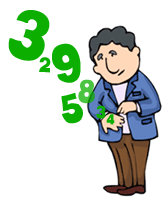# Three-Two-One GoooIf $a^{b^c}=6561$, then find the smallest possible value of $(a \times b \times c)$ such that $a,b,c$ are all integers.

×